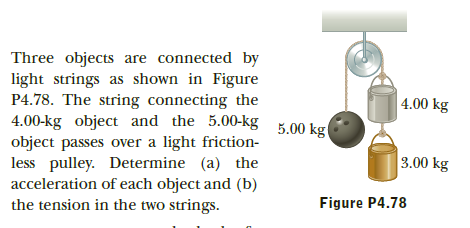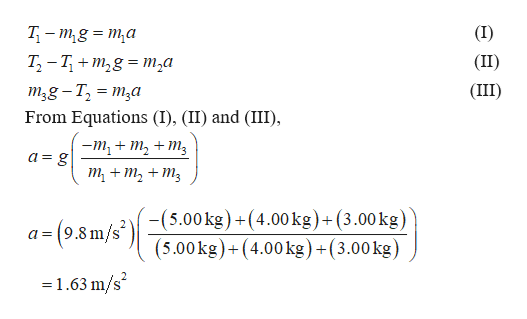# Three objects are connected bylight strings as shown in FigureP4.78. The string connecting the4.00-kg object and the 5.00-kg 5.00 kgobject passes over a light friction-less pulley. Determine (a) theacceleration of each object and (b)the tension in the two strings.| 4.00 kg3.00 kgFigure P4.78

Question
27 viewshelp_outlineImage TranscriptioncloseThree objects are connected by light strings as shown in Figure P4.78. The string connecting the 4.00-kg object and the 5.00-kg 5.00 kg object passes over a light friction- less pulley. Determine (a) the acceleration of each object and (b) the tension in the two strings. | 4.00 kg 3.00 kg Figure P4.78 fullscreen
check_circle

Step 1

The acceleration of the ma...help_outlineImage TranscriptioncloseТ - т,g %3D та T, -T, + m,g = m,a (I) (II) m2g - T, = m,a (III) From Equations (I), (II) and (III), — т, + т, + т; a = g т + т, + m, -(5.00kg)+(4.00kg)+(3.00 kg) (5.00 kg)+(4.00kg)+(3.00kg) a = (9.8 m/s') =1.63 m/s fullscreen

### Want to see the full answer?

See Solution

#### Want to see this answer and more?

Solutions are written by subject experts who are available 24/7. Questions are typically answered within 1 hour.*

See Solution
*Response times may vary by subject and question.
Tagged in

### Physics